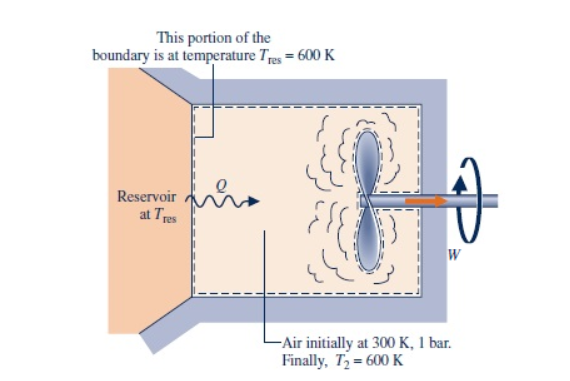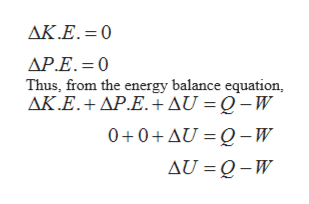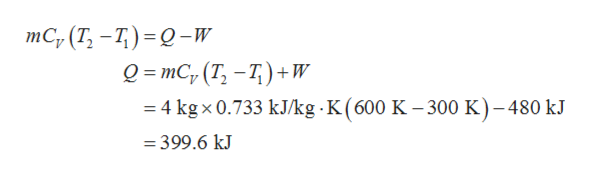# This portion of theboundary is at temperature Tres 600 KReservoirat TresW- Air initially at 300 K, 1 bar.Finally, T2 600 K

Question
2 views
A closed, rigid tank contains 4 kg of air initially at 300 K, 1 bar. As illustrated in the figure below, the tank is in contact with a thermal reservoir at 600 K and heat transfer occurs at the boundary where the temperature is 600 K. A stirring rod transfers W = -480 kJ of energy as shown in the diagram.The final temperature is 600 K. The air can be modeled as an ideal gas with cν = 0.733 kJ/kg · K and kinetic and potential energy effects are negligible.

Determine the amount of entropy transferred into the air and the amount of entropy produced, each in kJ/K.

 Q/Tb = kJ/K σ = kJ/Khelp_outlineImage TranscriptioncloseThis portion of the boundary is at temperature Tres 600 K Reservoir at Tres W - Air initially at 300 K, 1 bar. Finally, T2 600 K fullscreen
check_circle

Step 1

Consider ΔU as the change in internal energy, Q as the heat, and W as the work. The kinetic energy (K.E.) and potential energy (P.E.) are neglected. So,help_outlineImage TranscriptioncloseAK.E.= 0 AP.E. 0 Thus, from the energy balance equation, AK.E.+AP.E.AU = Q -W 0+0+AU = Q-W AU Q-W fullscreen
Step 2

Now, consider m as the mass, CV as the specific heat at constant volume, T2 and T1 as the final and initial temperature respectively. Thus, from the above equation, calculate the value of Q.help_outlineImage TranscriptioncloseтC, (T, —7) - 0-w о- тC, (T, —Т)+w 4 kg x 0.733 kJ/kg K ( 600 K - 300 K)-480 kJ =399.6 kJ fullscreen
Step 3

Consider Tb as the boundary temperature. Calculate the...

### Want to see the full answer?

See Solution

#### Want to see this answer and more?

Solutions are written by subject experts who are available 24/7. Questions are typically answered within 1 hour.*

See Solution
*Response times may vary by subject and question.
Tagged in

### Thermodynamics